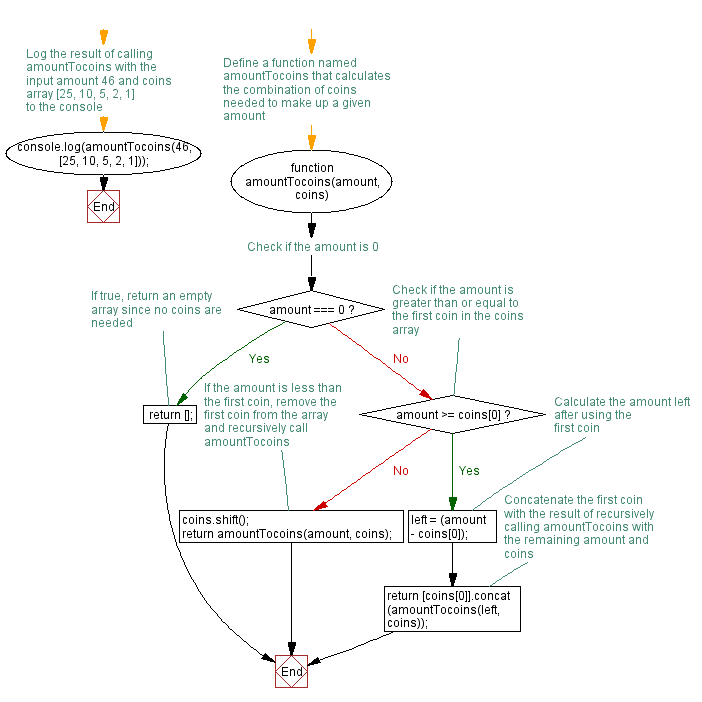# JavaScript: Convert an amount to coins

## JavaScript Function: Exercise-14 with Solution

Write a JavaScript function to convert an amount to coins.

Sample function : amountTocoins(46, [25, 10, 5, 2, 1])
Here 46 is the amount. and 25, 10, 5, 2, 1 are coins.
Output : 25, 10, 10, 1

Sample Solution: -

HTML Code:

``````<!DOCTYPE html>
<html>
<meta charset=utf-8 />
<title>Write a JavaScript function to convert an amount to coins.</title>
<body>

</body>
</html>
```
```

JavaScript Code:

``````function amountTocoins(amount, coins)
{
if (amount === 0)
{
return [];
}
else
{
if (amount >= coins)
{
left = (amount - coins);
return [coins].concat( amountTocoins(left, coins) );
}
else
{
coins.shift();
return amountTocoins(amount, coins);
}
}
}
console.log(amountTocoins(46, [25, 10, 5, 2,1]));
```
```

Sample Output:

```[25,10,10,1]
```

Flowchart:Live Demo:

See the Pen JavaScript - Convert an amount to coins-function-ex- 14 by w3resource (@w3resource) on CodePen.

Improve this sample solution and post your code through Disqus

What is the difficulty level of this exercise?

﻿

## JavaScript: Tips of the Day

JavaScript: Tagged template literals

```function getPersonInfo(one, two, three) {
console.log(one);
console.log(two);
console.log(three);
}

const person = 'Lydia';
const age = 21;

getPersonInfo`\${person} is \${age} years old`;
```

If you use tagged template literals, the value of the first argument is always an array of the string values. The remaining arguments get the values of the passed expressions!

Ref: https://bit.ly/323Y0P6